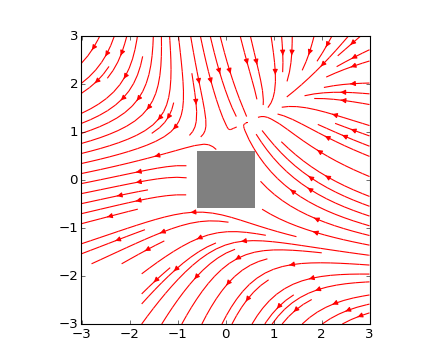### We're updating the default styles for Matplotlib 2.0

Learn what to expect in the new updates#### Previous topic

images_contours_and_fields example code: streamplot_demo_features.py

#### Next topic

lines_bars_and_markers Examples```"""
Demo of the streamplot function with masking.

This example shows how streamlines created by the streamplot function skips
"""
import numpy as np
import matplotlib.pyplot as plt

w = 3
Y, X = np.mgrid[-w:w:100j, -w:w:100j]
U = -1 - X**2 + Y
V = 1 + X - Y**2
speed = np.sqrt(U*U + V*V)